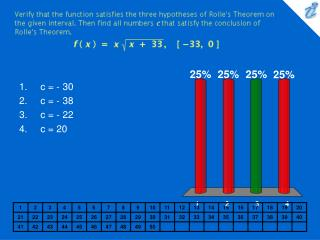DownloadDownload Presentationc = - 30 c = - 38 c = - 22 c = 20

# c = - 30 c = - 38 c = - 22 c = 20

Download Presentation## c = - 30 c = - 38 c = - 22 c = 20

- - - - - - - - - - - - - - - - - - - - - - - - - - - E N D - - - - - - - - - - - - - - - - - - - - - - - - - - -
##### Presentation Transcript

1. Verify that the function satisfies the three hypotheses of Rolle's Theorem on the given interval. Then find all numbers c that satisfy the conclusion of Rolle's Theorem. {image} • c = - 30 • c = - 38 • c = - 22 • c = 20

2. 1. 2. 3. 4. Find the exact values of the numbers c that satisfy the conclusion of The Mean Value Theorem for the function f ( x ) = x 3 - 2x for the interval [ - 2, 2 ]. • {image} • {image} • {image} • {image}

3. 1. 2. 3. 4. Verify that the function satisfies the hypotheses of The Mean Value Theorem on the given interval. Then find all numbers c that satisfy the conclusion of The Mean Value Theorem. {image} • {image} • {image} • {image} • {image}

4. 1. 2. 3. 4. If f ( 3 ) = 11 and {image} for {image} , how small can f ( 9 ) be? • {image} • {image} • {image} • {image}

5. 1. 2. 3. 4. At 5:00 P.M. a car's speedometer reads 15 mi/h. At 5:15 it reads 79 mi/h. At some time between 5:00 and 5:15 the acceleration is exactly x mi / {image} . Find x. • x = 270 mi / h2 • x = 278 mi / h2 • x = 263 mi / h2 • x = 256 mi / h2